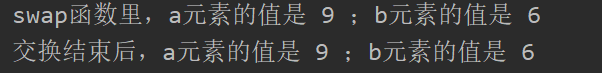• 列表元素交换位置
千次阅读
2020-02-09 23:22:09

# 1. 两个元素互换位置

a = [1,2]
print(a)
a, a = a, a
print(a)


列表是可变对象，变动里面的元素本身的id不会发生变化，以下代码说明了问题。

oneSimpleList = [1,2]
a = oneSimpleList
print(id(a), id(oneSimpleList))
print(a, oneSimpleList)

a, a = a, a

print(a, oneSimpleList)
print(id(a), id(oneSimpleList))


# 2. 四个元素互换位置

a = [1,2,3,4]
print(a)
a, a, a, a = a, a, a, a
print(a)


更多相关内容
• # 下面代码实现dw的a、b两个元素的值交换 dw['a'], dw['b'] = dw['b'], dw['a'] print("swap函数里，a元素的值是",dw['a'], "；b元素的值是", dw['b']) dw = {'a': 6, 'b': 9} swap(dw) print("交换结束后，a...

### 一、发现问题

今天研究python引用传递，发现了这么个例子：

def swap(dw):
# 下面代码实现dw的a、b两个元素的值交换
dw['a'], dw['b'] = dw['b'], dw['a']
print("swap函数里，a元素的值是",dw['a'], "；b元素的值是", dw['b'])

dw = {'a': 6, 'b': 9}

swap(dw)
print("交换结束后，a元素的值是", dw['a'], "；b元素的值是", dw['b'])



结果如下图：百思不得其解啊，你这怎么就交换了，让人匪夷所思啊

### 二、解决问题

经过查阅，发现，python底层是这么个情况：

x1,x2 = x2,x1的含义是： a = x2 b = x1 x1 = a x2 = b

那么就好理解了，dw[‘a’], dw[‘b’] = dw[‘b’], dw[‘a’] 就相当于如下代码：

    x = dw['a']
y = dw['b']
dw['b'] = x
dw['a'] = y


问题迎刃而解

展开全文python
• 今天小编就为大家分享一篇python 实现交换两个列表元素位置示例，具有很好的参考价值，希望对大家有所帮助。一起跟随小编过来看看吧
• 主要介绍了jQuery 实现DOM元素拖拽交换位置，本文通过实例代码给大家介绍的非常详细，对大家的学习或工作具有一定的参考借鉴价值,需要的朋友可以参考下
• jquery插件：点击上移、下移来交换li的位置。。。。。。jquery
• 看起来Python编译器使用这个结构优化了临时元组：码：import disdef swap1():a=5b=4a, b = b, adef swap2():a=5b=4c = aa = bb = cprint 'swap1():'dis.dis(swap1)print 'swap2():'dis.dis(swap2)输出：swap1():6 0 ...

看起来Python编译器使用这个结构优化了临时元组：

码：

import dis

def swap1():

a=5

b=4

a, b = b, a

def swap2():

a=5

b=4

c = a

a = b

b = c

print 'swap1():'

dis.dis(swap1)

print 'swap2():'

dis.dis(swap2)

输出：

swap1():

6 0 LOAD_CONST 1 (5)

3 STORE_FAST 0 (a)

7 6 LOAD_CONST 2 (4)

9 STORE_FAST 1 (b)

8 12 LOAD_FAST 1 (b)

15 LOAD_FAST 0 (a)

18 ROT_TWO

19 STORE_FAST 0 (a)

22 STORE_FAST 1 (b)

25 LOAD_CONST 0 (None)

28 RETURN_VALUE

swap2():

11 0 LOAD_CONST 1 (5)

3 STORE_FAST 0 (a)

12 6 LOAD_CONST 2 (4)

9 STORE_FAST 1 (b)

13 12 LOAD_FAST 0 (a)

15 STORE_FAST 2 (c)

14 18 LOAD_FAST 1 (b)

21 STORE_FAST 0 (a)

15 24 LOAD_FAST 2 (c)

27 STORE_FAST 1 (b)

30 LOAD_CONST 0 (None)

33 RETURN_VALUE

两个负载，一个ROT_TWO，两个保存，与三个负载和三个保存。你不太可能找到一个更快的机制。

展开全文• 怎么查找python列表元素位置Python中查找list中某个固定元素是常有的事，对于两种不同的查找有两种不同的解决方案，见下。 查找元素首次出现的位置：list自带的方法index可以找出某元素在list中首次出现的位置，...

怎么查找python列表中元素的位置

Python中查找list中某个固定元素是常有的事，对于两种不同的查找有两种不同的解决方案，见下。 查找元素首次出现的位置：list自带的方法index可以找出某元素在list中首次出现的位置，用法为： 其中，start为开始搜索的位置。

python 判断某个列表中的所有元素在另一个列表中

python类中两个列表实例如何相加或相减你若怀揣梦想，就永远不要允许自己只是试试”。

import numpy a = [1, 2, 3, 4] b = [5, 6, 7, 8] a_array = numpy.array(a) b_array = numpy.array(b) c_array = a_array + b_array d_array = a_array - b_array print c_array print d_array 扩展资料： 算术运算结果的数字类型与运算数的类

python 调整列表元素顺序

有一个没有重复单元的python列表，比如我想把列表第三个元素位置调整到a = [1, 2, 3, 4]item = a.pop(2) # 弹出第三个元素a.insert(0, item) # 作为第一个元素插入

python把列表前几个元素提取到新列表

我有个list有三十六个字符，我要把前n个字符转为数字，并且得到他们的和python把列表前几个元素提取到新列表步骤如下：

打开python语言命令窗口，定义一个列表变量C并进行赋值。

python list找出一个元素的位置(重复元素怎么分别使用list的index方法可以找到list中第一次出现该元素的位置 >>> l = ['a','b','c','c','d','c']>>> find='b'>>> l.index(find)1找出出现该元素的所有位置可以使用一个简单的表理解来实现 >>> find = 'c'>>> [i for i,v in enumerate(l) if v==fi

如何在python列表中查找某个元素的索引

方法二：利用enumerate函数。什么都不怕，就怕晴川不愿嫁，八哥听了到地又散架。

python3 如何将一个列表内指定的两个元素按他们出比如a=[1,2,3,4,2,1,1,4,5] 怎么将1和2按他们在列表a中的顺序添加到新的b = []a=[1,2,3,4,2,1,1,4,5]for i in a: if i == 1 or i == 2: b.append(i)

python用户输入一个列表和两个整数作为下标然后使Python 3 的 input() 函数相当于 Python 2 的 raw_input() 函数。 # 输入数字 # Python 3 通过 input() 获取的任何内容都是 str(字符串)类型。 str1 = input('请输入第一个数：') str2 = input('请输入第二个数：') # 用 int() 函数强制将 str

展开全文• 99999,20,3,50]需求：将列表中最大值与最小值分别放至列表第一和最后一位于是执行以下操作：list,list[list.index(max(list))]=list[list.index(max(list))],list打印结果print(list)结果如下：将中间交换的...
• I have a list and I need to swap the 1st element in the list with the maximum element in the list.But why does code 1 work while code 2 doesn't:code 1:a = list.index(max(list))list, list[a] = list...
• 对List中的元素调换位置，将data中的index1与index2元素互换位置 //data 为List Collections.swap(data,index1,index2);java
• 代码】Python：将列表指定位置的两个元素调换位置python
• import java.util.Collections;.../*** 集合工具类* 向前移动问题：把第一个元素与第三个元素调换一下* 向后移动问题：把第三个元素与第一个元素调换一下* Created by crystal on 2017/6/9.*/public class Col...
• 在IDLE 中验证如下： >>> numbers = [5, 6, 7] >>> i = 0 >>> numbers[i], numbers[i+1] = numbers[i+1], numbers[i] >>> numbers [6, 5, 7] 最后你的问题表述【交换两个列表元素位置】改成【交换列表中两个元素...
• 今天做了个 两个元素互换位置 的动画 ，记录一下 首先 获得两个元素得绝对坐标定位 然后通过animation 实现移动 其他效果 按自己喜好添加 (function($){ ... //交换两个元素位置 //源绝对位置 va • 两个元素位置互换 change_list = [6, 8] print(change_list) # 交换列表，以下两个均可 # change_list, change_list = change_list, change_list change_list, change_list = change_list, ...列表list • python 中交换两个列表元素位置 >>> numbers = [5, 6, 7] >>> i = 0 >>> numbers[i], numbers[i+1] = numbers[i+1], numbers[i] >>> numbers [6, 5, 7] ... • C# list 交换元素位置 private static List<T> Swap<T>(List<T> list, int index1, int index2) { var temp = list[index1]; list[index1] = list[index2]; list[index2] = temp; return list... • 定义一个方法swap()传入集合和两个角标使集合中元素交换位置 使用创建第三方变量的方式实现两个元素的位置交换 // An highlighted block public static void main(String[] args) { List<String> list=new ... • <div ondrop="drop(event,this)" ondragover="allowDrop(event)...拖拽元素1</div> <div ondrop="drop(event,this)" ondragover="allowDrop(event)" draggable="true" ondragstart="drag(event, this)"> • 1.交换位置 const list1 = [ { labelId: 0, name: 'xiaomin' }, { labelId: 1, name: 'xiaohong' }, ]; const list2 = [ { labelId: 0, name: 'xiaomin' }, { labelId: 1, name: 'xiaohong' },... • //交换2和4的位置 const temp = arr.splice(3, 1, arr);//先将2插入到4的位置，并且覆盖掉4，此时temp能拿到4，此时temp为，此时的arr为[1,2,3,2,5] arr = temp; // 将为2替换成4 consreactjs • ——————————————————————————————————————————code:member=[‘文殊‘,‘普贤‘,‘观音‘,‘弥勒‘]print(‘调换前的列表‘)print(member)#调换列表的前两个元素temp=... • a = input("请输入a:") b = input("请输入b:")print("a = %s;b = %s"%(a,b)) c = a ##先把 老王 放到 仓库 a = b ##在把 老铁 放到 老王的位置 b = c ##从仓库中 把老王 放到 老铁 中print("a = %s;b = %s"%(a,b))python • Python实现判断并移除列表指定位置元素的方法本文实例讲述了Python实现判断并移除列表指定位置元素的方法。分享给大家供大家参考，具体如下：问题很简单，输入一个列表和索引，若索引超出列表范围则返回源列表，否则... • i和i+1.交换相当于是i后移一位 var tempOption = this.resourceLists[i+1]; this.$set(this.resourceLists, i+ 1, this.resourceLists[i]);  this.\$set(this.resourceLists, i,...
• 这个练习，主要考察的是关于列表下标及下标元素的交换练习。文艺.jpg总体来说，还不算特别复杂网上同样也查了三处资料，原出处的代码量太过庞杂，...# 输入数组，最大的与第一个元素交换，最小的与最后一个元素交...
• Python既具有普通程序开发语言的特点，也具有Matlab语言用于数值计算的特点，，当然了数值计算是由其其强大的第三方库numpy实现的,矩阵在python中数据类型是ndarray，python中交换两个数值的代码和交换同一矩阵......